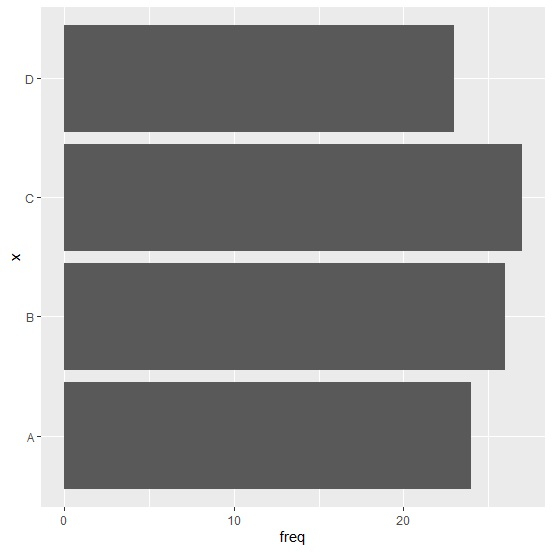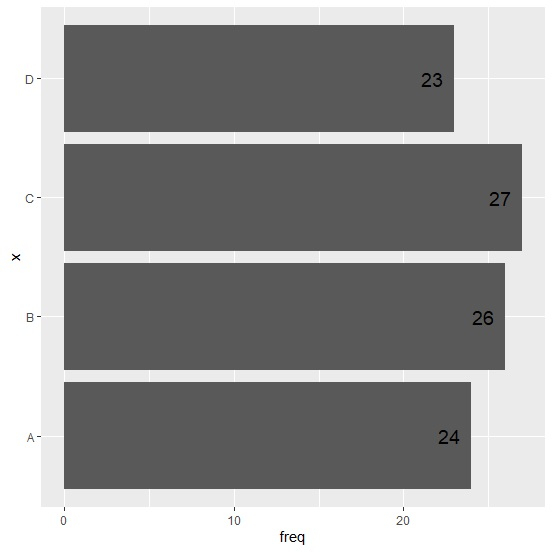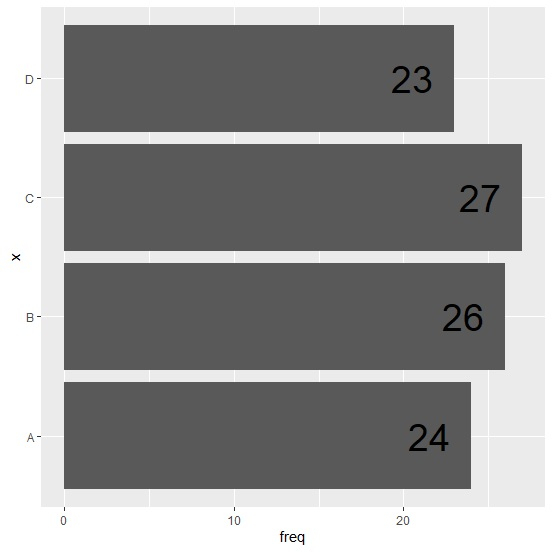# How to create a horizontal bar chart using ggplot2 with labels at inside end of the bars in R?

R ProgrammingServer Side ProgrammingProgramming

To create a horizontal bar chart using ggplot2 package, we need to use coord_flip() function along with the geom_bar and to add the labels geom_text function is used. These two functions of ggplot2 provides enough aesthetic characteristics to create the horizontal bar chart and put the labels at inside end of the bars.

## Example

Live Demo

x<-c("A","B","C","D")
freq<-c(24,26,27,23)
df<-data.frame(x,freq)
df

## Output

x freq
1 A 24
2 B 26
3 C 27
4 D 23
library(ggplot2) ggplot(df,aes(x,freq))+geom_bar(stat="identity")+coord_flip()

## Output> ggplot(df,aes(x,freq,label=freq))+geom_bar(stat="identity")+geom_text(size=5,hjust=1.5)+coord_flip()

## Output> ggplot(df,aes(x,freq,label=freq))+geom_bar(stat="identity")+geom_text(size=10,hjust=1.5)+coord_flip()

## Output